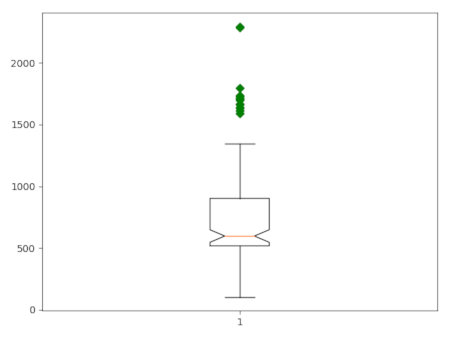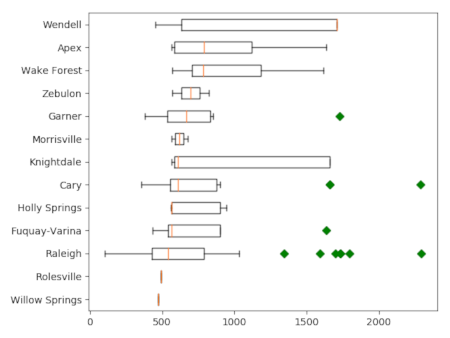## NAME

d.vect.colbp - Draws the boxplot of values in a vector attribute column

## KEYWORDS

display, vector, plot, histogram, boxplot

## SYNOPSIS

d.vect.colbp
d.vect.colbp --help
d.vect.colbp [-honr] map=name [layer=string] column=name [where=sql_query] [plot_output=name] [group_by=name] [order=string] [--overwrite] [--help] [--verbose] [--quiet] [--ui]

### Flags:

-h
horizontal boxplot(s)
Draw the boxplot horizontal
-o
hide outliers
Draw boxplot(s) without outliers
-n
notch
Draw boxplot(s) with notch
-r
Rotate labels
rotate x-axis labels
--overwrite
Allow output files to overwrite existing files
--help
Print usage summary
--verbose
Verbose module output
--quiet
Quiet module output
--ui
Force launching GUI dialog

### Parameters:

map=name [required]
Name of vector map
Or data source for direct OGR access
layer=string
Layer number or name
Vector features can have category values in different layers. This number determines which layer to use. When used with direct OGR access this is the layer name.
Default: 1
column=name [required]
Attribute column value to be plotted
where=sql_query
WHERE conditions of SQL statement without 'where' keyword
Example: income < 1000 and population >= 10000
plot_output=name
Name for output file
group_by=name
Attribute column with categories to group the data by
order=string
Sort boxplots
Sort boxplots based on their median values
Options: descending, ascending

## DESCRIPTION

d.vect.colbp draws a boxplot of the values in a vector map attribute column. The user can use the where option to only select a subset of the attribute table. There is also the option to group the values of the column according to the categories in a second column (group_by and create one plot with for each group a separate boxplot.

By default, the resulting plot is displayed on screen (default). However, the user can also save the plot to file using the plot_output option. The format is determined by the extension given by the user. So, if plot_output = outputfile.png, the plot will be saved as a png file.

There are a few additonal layout options, including the option to rotate the plot and the x-axis labels. Furthermore, the user can optinally plot the boxplot(s) with notches and without outliers.

## NOTE

This script, based on d.vect.colhist, is a quick and dirty solution sing basic matplotlib, and will need some further fine-tuning and testing.

## EXAMPLE

### Example 1

Use the vector layer schools_wake from the NC sample dataset to create boxplots of the core capacity of the schools in Wake County, North Carolina. Use the Where clause to exclude all records with no data.
```d.vect.colbp -n map=schools_wake column=CORECAPACI where="CORECAPACI >0"
```Figure 1: Boxplot of core capacity of schools in Wake County.

### Example 2

Use the vector layer schools_wake from the NC sample dataset to create boxplots of the core capacity of the schools in Wake County, North Carolina, grouped by city. Use the Where clause to exclude all records with no data.
```d.vect.colbp -h --overwrite map=schools_wake column=CORECAPACI where="CORECAPACI >0" group_by=ADDRCITY order=ascending
```Figure 2" Boxplot of core capacity of schools in Wake County, grouped by city.

## AUTHOR

Paulo van Breugel
Based on the d.vect.colhist addon by Moritz Lennert

## SOURCE CODE

Available at: d.vect.colbp source code (history)

© 2003-2021 GRASS Development Team, GRASS GIS 7.8.7dev Reference Manual